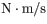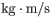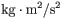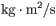# Problem: Which of the following is the SI unit of angular momentum?a.b.c.d.

###### FREE Expert Solution

Angular momentum:

$\overline{){\mathbf{L}}{\mathbf{=}}{\mathbf{I}}{\mathbf{\omega }}}$

I is the moment of inertia and ω is the moment of inertia.

Angular velocity:

$\overline{){\mathbf{\omega }}{\mathbf{=}}\frac{\mathbf{v}}{\mathbf{r}}}$

96% (24 ratings)###### Problem Details

Which of the following is the SI unit of angular momentum?

 a.b.c.d.